### Graphs Class 10th Mathematics Tamilnadu Board Solution

##### Question 1.A bus travels at a speed of 40 km/hr. Write the distance-time formula and draw the graph of it. Hence, find the distance travelled in 3 hours.Answer:Suppose a body X travels a at a speed of S km/h for T hours then the distance covered by him will be D and its given asD = ST kmHere speed of bus, s = 40 km/hrTravel time , t = 3hrsSo using this we can find a set of distance and put them in graph to find the exact distance travelled by the bus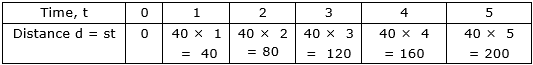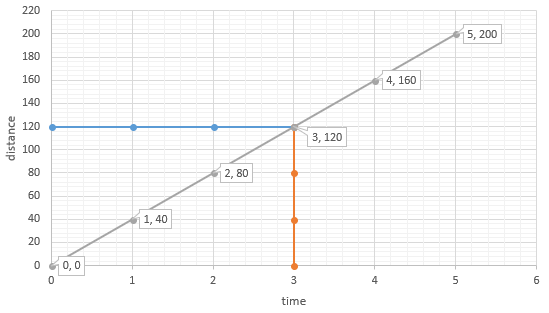to know the distance covered by the bus at three hours draw a line parallel to Y-axis from X = 3 to the curve and from the intersecting point of that line and the curve draw another line parallel to X-axis and the point at which the line intersect the Y-axis will give the distance coveredso from the graph it’s clear that as the bus was running for 3 hrs. and it covered a distance of 120 km.Question 2.The following table gives the cost and number of notebooks bought.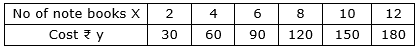Draw the graph and hence (i) find the cost of seven note books.(ii) How many note books can be bought for ₹165.Answer:From the above it’s clear that y = 15 × XPutting the above value in graph we will find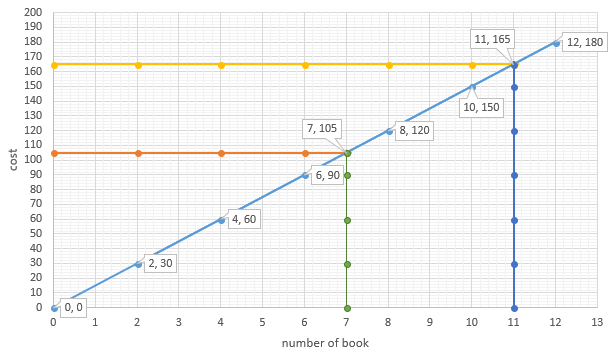(i) To find the cost of 7 books draw a line parallel to Y-axis from X = 7 to the curve and from the intersecting point of that line and the curve draw another line parallel to X-axis and the point at which the line intersect the Y-axis will give the cost of 7 books.so from the graph cost of 7 books is found to be ₹105(ii) to find the number of book that can be purchased at a cost of 165 draw a line parallel to X-axis from Y = 165.from the point at which the line intersect the curve draw another line parallel to Y-axis and the point at which the line intersect x-axis will give the number of book.so we can buy 11 note booksQuestion 3.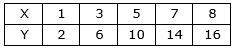Draw the graph for the above table and hence find(i) the value of y if x = 4(ii) the value of x if y = 12Answer:Using the data we can draw a graph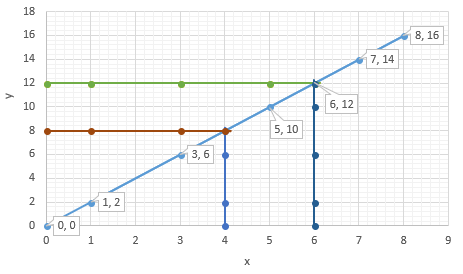(I) TO FIND THE VALUE OF the value of y if x = 4, draw a line parallel to Y-axis from X = 4 to the curve and from the intersecting point of that line and the curve draw another line parallel to X-axis and the point at which the line intersect the Y-axis will give the value of Y ay X = 4.so from the graph at X = 4 Y is found to be 8(II) to find the value of X at Y = 12 draw a line parallel to X-axis from Y = 12 and from the point at which the line intersect the curve draw another line parallel to Y-axis and the point at which the line intersect x-axis will give the value of X.so we find at Y = 12 Xis found to be 6Question 4.The cost of the milk per liter is ₹15. Draw the graph for the relation between the quantity and cost. Hence find(i) the proportionality constant.(ii) the cost of 3 litres of milk.Answer:(i) k = 15 (ii) ₹45Let the cost of milk be Y and X be the quantity of milk in liter. As it’s given that cost per one liter is 15rs so increase in quantity will increase the cost of milk. So it’s a direct proportionMathematically,Y = KXWhere K is the constant of proportionalitySo we can draw a table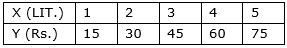so from the graph it’s clear that y = kx = 15xProportionality, k = 15We can draw a graph using the table given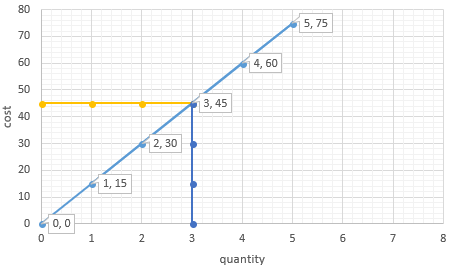To find the cost of 3 liter milk we have to draw a line parallel to Y-axis at X = 3 then from the intersection point of that parallel line and the curve draw another line parallel to X-axis so the point of intersection of that line and Y-axis will give the cost of 3 liter of milk.From the graph its clear that cost of 3 liter is 45 RS.Question 5.Draw the Graph of xy = 20, x, y > 0. Use the graph to find y when x = 5, and to find x when y = 10.Answer:We are given that xy= 20⇒ y = (20/x)We can find different value of Y by taking values of x between 1 and 10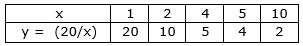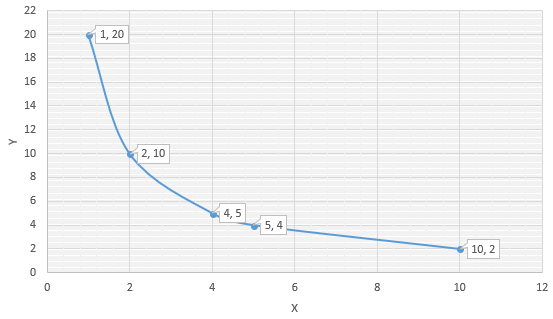From the graph we get that at x = 5, y = 4, and to find y = 10, x = 2Question 6.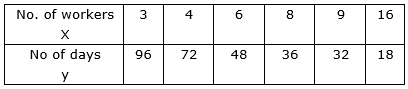Draw graph for the data given in the table. Hence find the number of days taken by 12 workers to complete the work.Answer:We can construct a graph using the data given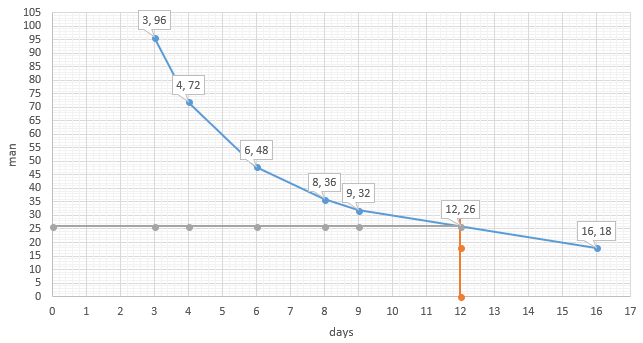To find the number of days taken by 12 worker to complete the work draw a line parallel to Y-axis from X = 12 to the curve and from the intersecting point of that line and the curve draw another line parallel to X-axis and the point at which the line intersect the Y-axis will give the value of Y at X = 12.so from the graph at X = 12 Y is found to be 26

PDF FILE TO YOUR EMAIL IMMEDIATELY PURCHASE NOTES & PAPER SOLUTION. @ Rs. 50/- each (GST extra)

HINDI ENTIRE PAPER SOLUTION

MARATHI PAPER SOLUTION

SSC MATHS I PAPER SOLUTION

SSC MATHS II PAPER SOLUTION

SSC SCIENCE I PAPER SOLUTION

SSC SCIENCE II PAPER SOLUTION

SSC ENGLISH PAPER SOLUTION

SSC & HSC ENGLISH WRITING SKILL

HSC ACCOUNTS NOTES

HSC OCM NOTES

HSC ECONOMICS NOTES

HSC SECRETARIAL PRACTICE NOTES

2019 Board Paper Solution

HSC ENGLISH SET A 2019 21st February, 2019

HSC ENGLISH SET B 2019 21st February, 2019

HSC ENGLISH SET C 2019 21st February, 2019

HSC ENGLISH SET D 2019 21st February, 2019

SECRETARIAL PRACTICE (S.P) 2019 25th February, 2019

HSC XII PHYSICS 2019 25th February, 2019

CHEMISTRY XII HSC SOLUTION 27th, February, 2019

OCM PAPER SOLUTION 2019 27th, February, 2019

HSC MATHS PAPER SOLUTION COMMERCE, 2nd March, 2019

HSC MATHS PAPER SOLUTION SCIENCE 2nd, March, 2019

SSC ENGLISH STD 10 5TH MARCH, 2019.

HSC XII ACCOUNTS 2019 6th March, 2019

HSC XII BIOLOGY 2019 6TH March, 2019

HSC XII ECONOMICS 9Th March 2019

SSC Maths I March 2019 Solution 10th Standard11th, March, 2019

SSC MATHS II MARCH 2019 SOLUTION 10TH STD.13th March, 2019

SSC SCIENCE I MARCH 2019 SOLUTION 10TH STD. 15th March, 2019.

SSC SCIENCE II MARCH 2019 SOLUTION 10TH STD. 18th March, 2019.

SSC SOCIAL SCIENCE I MARCH 2019 SOLUTION20th March, 2019

SSC SOCIAL SCIENCE II MARCH 2019 SOLUTION, 22nd March, 2019

XII CBSE - BOARD - MARCH - 2019 ENGLISH - QP + SOLUTIONS, 2nd March, 2019

HSC Maharashtra Board Papers 2020

(Std 12th English Medium)

HSC ECONOMICS MARCH 2020

HSC OCM MARCH 2020

HSC ACCOUNTS MARCH 2020

HSC S.P. MARCH 2020

HSC ENGLISH MARCH 2020

HSC HINDI MARCH 2020

HSC MARATHI MARCH 2020

HSC MATHS MARCH 2020

SSC Maharashtra Board Papers 2020

(Std 10th English Medium)

English MARCH 2020

HindI MARCH 2020

Hindi (Composite) MARCH 2020

Marathi MARCH 2020

Mathematics (Paper 1) MARCH 2020

Mathematics (Paper 2) MARCH 2020

Sanskrit MARCH 2020

Sanskrit (Composite) MARCH 2020

Science (Paper 1) MARCH 2020

Science (Paper 2)

MUST REMEMBER THINGS on the day of Exam

Are you prepared? for English Grammar in Board Exam.

Paper Presentation In Board Exam

How to Score Good Marks in SSC Board Exams

Tips To Score More Than 90% Marks In 12th Board Exam

How to write English exams?

How to prepare for board exam when less time is left

How to memorise what you learn for board exam

No. 1 Simple Hack, you can try out, in preparing for Board Exam

How to Study for CBSE Class 10 Board Exams Subject Wise Tips?

JEE Main 2020 Registration Process – Exam Pattern & Important Dates

NEET UG 2020 Registration Process Exam Pattern & Important Dates

How can One Prepare for two Competitive Exams at the same time?

8 Proven Tips to Handle Anxiety before Exams!